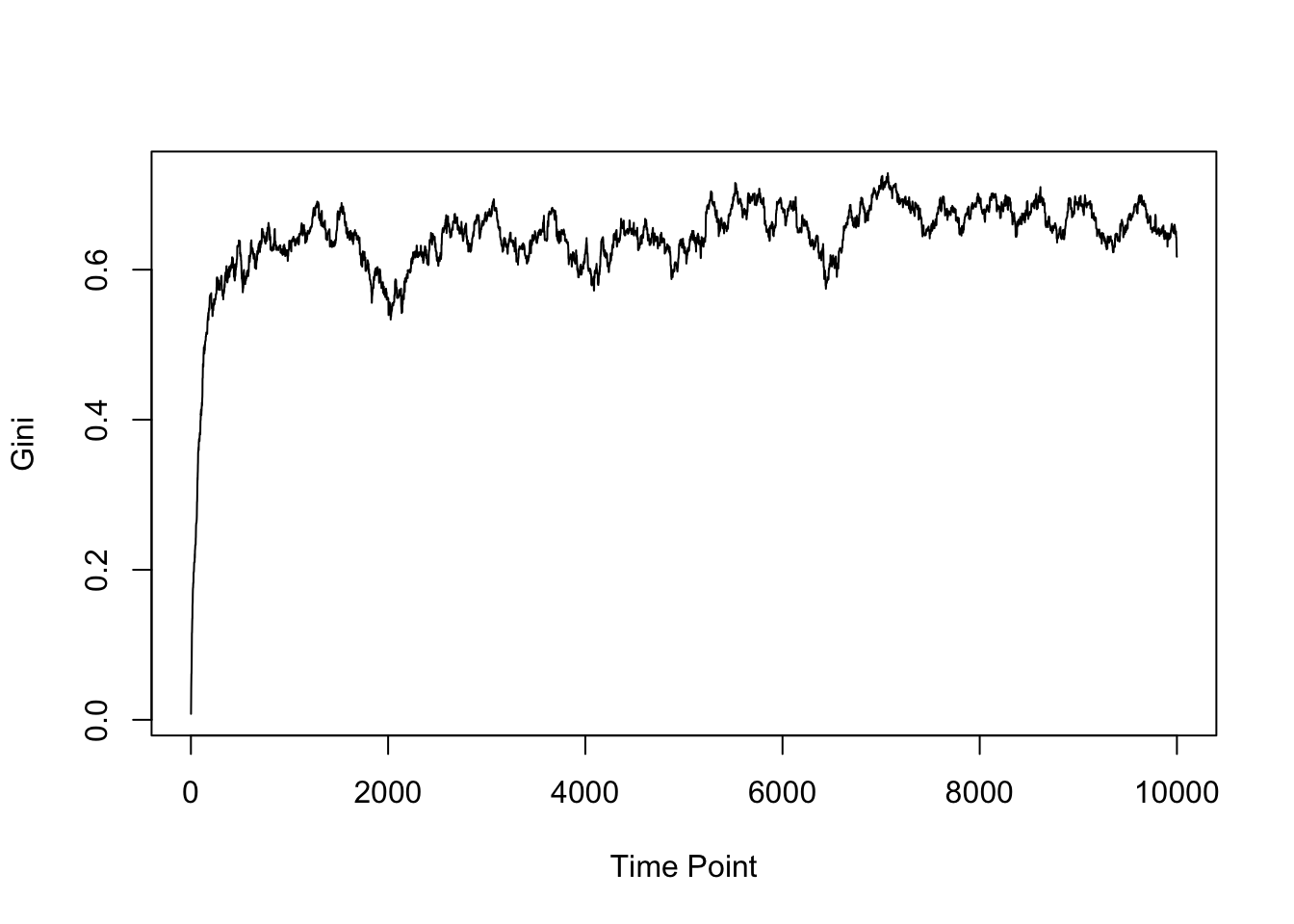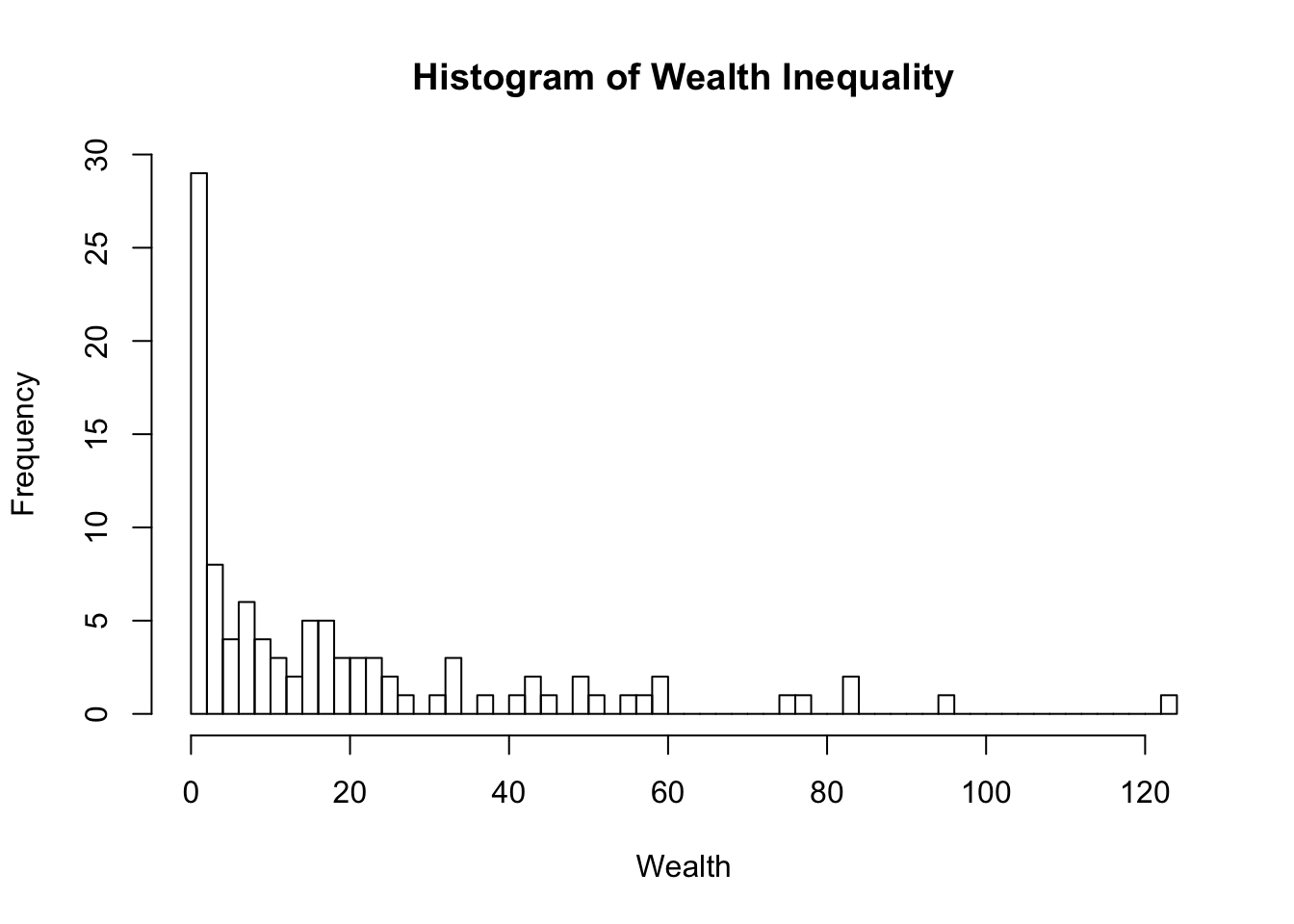This is a quick demo of what I think is a good early programming assignment in R, something that should shortly follow after “FizzBuzz.” It is very loosely based off of the economic ideas of C. Wright Mills (Renzulli, Aldrich, and Reynolds 2003). The idea is that wealth inequality can be modeled as a natural phenomenon. If a group of people start out with the same amount of money and then begin exchanging money at random, eventually most of the money ends up in the hands of a few people. There are a few ways to visualize this process in the economy. Besides showing statistics like the change in average, median, or maximum amount of wealth over time, it’s also a good opportunity to create a function to track the Gini coefficient for the population over time. This exercise combines several important R programming concepts: objects, writing functions, simulations, randomization, and visualization.

set.seed(2018-01-22)

# x is a vector of numbers.
# This function returns a single number, the Gini coefficient of the vector.
# A higher Gini means less equality between the numbers in the vector.
gini <- function(x){
num <- 0
for(i in seq_along(x)){
num <- num + sum(abs(x[i] - x[-i]))
}

num / (2 * length(x) * sum(x))
}

# There are 100 people in our economy, they each get \$10 to start.
people <- rep(10, 100)

# This vector will keep track of the Gini for the economy
# at each time point.
gini_snapshot <- rep(NA, 10000)

for(i in 1:10000){
# Choose two people in the economy at random.
a_ind <- sample(1:100, 1)
b_ind <- sample(1:100, 1)

# Find out how much money each person has.
a_money <- people[a_ind]
b_money <- people[b_ind]

# Choose a random number of dollars that person A will give to person B.
exchange <- sample(0:a_money, 1)

# Subtract that amount of money from A's account.
people[a_ind] <- a_money - exchange

# Add that amount of money to B's account.
people[b_ind] <- b_money + exchange

# Calculate Gini coefficient for this time point in the simulation.
gini_snapshot[i] <- gini(people)
}

plot(gini_snapshot, type = "l", xlab = "Time Point", ylab = "Gini")hist(people, breaks = 50, xlab = "Wealth",
main = "Histogram of Wealth Inequality")Renzulli, Linda A., Howard E. Aldrich, and Jeremy Reynolds. 2003. “It’s up in the Air, or Is It?” Teaching Sociology 31 (January): 49–59. http://www.asanet.org/sites/default/files/savvy/introtosociology/Documents/TSRenzulli2003.pdf.